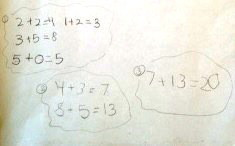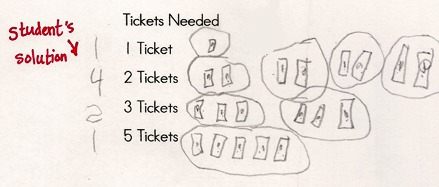# 20 Tickets

Alignments to Content Standards: 1.OA.A.1 1.OA.D.7

#### Materials

• 20 counters or linking cubes per pair of students
• pencil
• copy of the problem

#### Actions

The teacher poses the problem:

Bo bought 20 tickets to play games at Family Fun Night at his school. He wants to play each game at least once. He needs to use all of his tickets. How many times might he play each game? Find at least two ways he can do it.

Game Number of Tickets Needed
Ring Toss 1
Putt-Putt Golf 2
Soccer Kick 3
Moonwalk 5

When all pairs of students have had a chance to find at least one solution, the teacher can lead a whole-group discussion and record each solution as an equation on chart paper or the chalkboard/whiteboard/SmartBoard.

## IM Commentary

The purpose of the task is for students to add and subtract within 20 (1.OA.1) and represent complex addition problems with an equation to increase their understanding of and flexibility with the equals sign (1.OA.7). There are multiple solutions, and each pair of students should find more than one. The students can use the counters or linking cubes to represent each ticket needed to do each game, but then they should be encouraged to draw a picture to represent their work so there is a record of their thought process. Students who are comfortable with symbolic representations can record their solutions using equations.

The problem can be differentiated by using either a smaller or larger number of tickets. An extension would also be to have the students find the greatest number of times the games could be played to still do all games at least once. Another would be to ask if they can play each game twice and justify their thinking and solution.

The solutions shown below are based on actual student solutions.

The Standards for Mathematical Practice focus on the nature of the learning experiences by attending to the thinking processes and habits of mind that students need to develop in order to attain a deep and flexible understanding of mathematics. Certain tasks lend themselves to the demonstration of specific practices by students. The practices that are observable during exploration of a task depend on how instruction unfolds in the classroom. While it is possible that tasks may be connected to several practices, only one practice connection will be discussed in depth. Possible secondary practice connections may be discussed but not in the same degree of detail.

This particular task helps illustrate Mathematical Practice Standard 4, Model with mathematics. Students apply the mathematics they know to solve problems arising in everyday life. For this problem, young children might arrange concrete objects such as linking cubes or counters to represent the mathematical elements of the problem, draw a picture, or write an addition sentence to describe the situation. This problem is complex in that students will need to track the number of tickets needed for four different games. Each game requires a different amount of tickets and Bo must use all of his tickets. It doesn’t matter whether children use counters or linking cubes or draw pictures. The real significance lies in that students can identify the mathematical elements of the situation and represent them appropiately.

## Solutions

Solution: Using concrete objects

One solution is:

• 1 ring toss

• 3 Putt Putt Golf

• 1 Soccer Kick

• 2 Moonwalks Students can use linking cubes or counters to represent the required tickets.One equation that represents this is

$$1+0+5+5+2+3+2+2=20$$

Some students might also record their thinking using equations:Another equation that represents this is $$2+2+1+2+3+5+5+0=20$$

Solution: Starting with one of each

First, play each game once:

$$1+2+3+5=11$$

11 tickets are used. 20 - 11 = 9, so there are 9 tickets left.

One way to use the rest of the tickets is to play Ring Toss, Soccer Kick and Moonwalk again because $$1+3+5= 9$$

• Ring Toss: 2
• Putt-Putt Golf: 1
• Soccer Kick: 2
• Moonwalk: 2

We can write this solution as an equation in different ways:

$$1+1+2+3+3+5+5=20$$ or $$1+2+3+5+1+3+5=20$$ or $$2+2+6+10=21$$

Solution: Drawing picturesAn equation that represents this solution is $$1 + 2+2+2+2+3+3+5=20$$